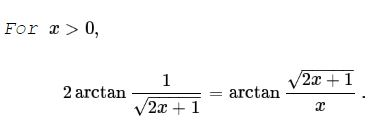# Arctan Identity and its Consequence

18 May 2016, Created with GeoGebra

### Explanation

Assume in a right $\Delta BCD,\;$ $AC\perp BD,\;$ with $A,E,F\;$ on $BC\;$ such that $DE=AB\;$ and $EF=AF.\;$ Then $\angle AFC=2\angle ACB.$### Statement### Proof 1

As is well known, $\displaystyle \tan 2\alpha=\frac{2\tan\alpha}{1-\tan^2\alpha}.\;$ Using that and $\tan(\arctan\alpha)=\alpha,$

\displaystyle\begin{align} \tan\left( 2\arctan\frac{1}{\sqrt{2x+1}}\right)&=\frac{\displaystyle\frac{2}{\sqrt{2x+1}}}1-\left(\frac{1}{\sqrt{2x+1}}\right)^2}\\ &=\frac{\displaystyle\frac{2}{\sqrt{2x+1}}}\frac{2x}{2x+1}}\\ &=\frac{\sqrt{2x+1}}{x}. \end{align

This proves the statement which can be rewritten as

$\displaystyle\frac{\displaystyle\arctan\frac{1}{\sqrt{2x+1}}}{\displaystyle\arctan\frac{\sqrt{2x+1}}{x}}=\frac{1}{2}.$

### Proof 2

Define $\displaystyle f(x)=2\arctan\frac{1}{\sqrt{2x+1}}\;$ and $\displaystyle g(x)=\arctan\frac{\sqrt{2x+1}}{x}.\;$ Then

$\displaystyle f'(x)=-\frac{1}{\sqrt{2x+1}(x+1)},\\ \displaystyle g'(x)=-\frac{1}{\sqrt{2x+1}(x+1)}.$

It follows that $f(x)-g(x)=\mbox{const}.\;$ But $\displaystyle f(1)=2\arctan\left(\frac{1}{\sqrt{3}}\right)=2\cdot\frac{\pi}{6},\;$ while $\displaystyle g(1)=\arctan\sqrt{3}=\frac{\pi}{3}.\;$ Hence $f(x)=g(x).$

### Corollary

$\displaystyle\sum_{k=1}^n\frac{\displaystyle\arctan\frac{1}{\sqrt{2k+1}}}{\displaystyle\arctan\frac{\sqrt{2k+1}}{k}}=\frac{n}{2}.$

### Acknowledgment

What I made as Corollary has been posted by Rovshan Pirkuliev at the Imad Zak Math facebook page. Proof 1, based on the Statement above, is due to Ghimisi Dumitrel. Proof 2 is by Imad Zak. The applet at the top of the page is just an illustration of the underlying trigonometric identity. This is certainly not the most efficient way to double an angle.

### Trigonometry